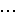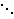# QR DecompositionSuppose we have a problem that can be modeled by the system of equations Ax = b with a matrix A and a vector b. We have already shown how to use Gaussian Elimination to solve these methods, but I would like to introduce you to another method – QR decomposition. In addition to solving the general system of equations, this method can also be used in a number of other algorithms including the linear regression analysis to solve the least squares problem and to find the eigenvalues of a matrix.

Generally, when we see a system of equations, the best way to proceed in solving it is Gaussian elimination, or LU decomposition. However, there are some very special matrices where this method can lead to rounding errors. In such cases, we need a better, or more stable algorithm, which is when the QR decomposition method becomes important.

Any matrix A, of dimensions m by n with m >= n, can be represented as the product of two matrices, an m by m orthogonal matrix Q, i.e. QTQ = I, and an m by n upper triangular matrix R with the form [R 0]T. We perform this decomposition by will first converting the columns of the matrix A into vectors. Then, for each vector ak, we will calculate the vectors uk and ek given by

uk =i = 1 to k-1projej ak
and
ek = uk / ||uk||
Then Q = [e1, …, en] and

R =00 0# Gaussian Elimination

I have just written a script which executes the Gaussian Elimination Algorithm.

When we have a collection of lines we wish to know if they all intersect at some point. Many times we are interested in determining what that point is. In order to calculate this information, we first need an understanding of the lines themselves. The way the Gaussian Elimination Algorithm works is that the collection of lines are input using a notation of Ax = b, where the matrix A is called the coefficient matrix, as the nth row of it corresponds to the coefficients for the nth line being considered. The vector b represents the right hand side vector (in two dimensions, we would call these constants the y-intercepts of the lines. In higher dimensions they hold a similar property). The vector x represents the point where the lines intersect. It is this quantity which Gaussian Elimination seeks to determine.

The basic procedure of Gaussian Elimination is to use \”elementary row operations\” on the matrix (A|b), which is called the augmented matrix, to transform A into upper triangular form. Once this is done, a procedure called back-substitution can find the solution (x) to this problem.

The elementary row operations that we are allowed to perform are:

• Interchange two rows.
• Multiply a row by a nonzero number.
• Add a row to another one multiplied by a number.
• For the last property listed above, we will determine this number by dividing the coefficient of the term we which to eliminate by the negative of the coefficient of the element on the main diagonal of the same column of the matrix. This will have the property of cancelling out, or producing a desired zero in the resulting row.

If this algorithm produces an upper triangular matrix from which we can solve for x using back-substitution. This procedure of back-substitution is simply solving for the vector x from the bottom of the matrix to the top. If the algorithm does not produce an upper triangular matrix (because somewhere along the line, we are unable to obtain a ratio because we have zero’s on the diagonal and all zeros below the diagonal), then we say the matrix is singular. This means that there is no unique point where the lines all intersect.

To learn more and see more examples, check out My Script on Gaussian Elimination.